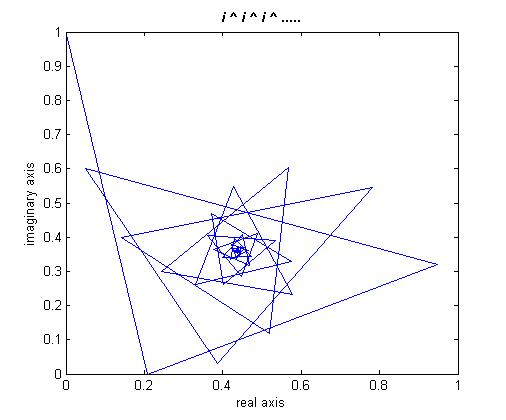# i ^ i ^ i ^ … ?

Today on the internet I saw the calculation for i^i, where i is the square root of -1. It can be solved pretty easily using Euler’s formula, where any number z in the complex plane can be expressed as
$$\textstyle{}$$

$$\displaystyle{z=r\,e^{i\,\theta}}$$

$$\displaystyle{}$$

where $$r$$ is the absolute value of the number, $$e = 2.718281828\ldots$$ is the base of the natural logarithm, $$i$$ is of course $$\sqrt{-1}$$, and $$\theta$$ is the angle in radians of the number measured counterclockwise from the positive real axis, essentially giving the complex number in polar coordinates. So for example $$1\,e^{\textstyle{i\:0}}=1$$, $$1\,e^{\textstyle{i\:\pi}}=1$$, $$1\,e^{\textstyle{i\frac{\pi}{2}}}=i$$, etc. We know that $$i^i$$ is going to be some number in the complex plane, so we can express that number as $$\textstyle{r\,e^{i\,\theta}=e^{\text{ln}(r)}e^{i\,\theta}}= e^{\text{ln}(r)+i\,\theta}=e^z$$, where $$z=\text{ln}(r)+i\,\theta$$ is itself a complex number.

This gives us an equation
$$\displaystyle{i^i = e^z}$$

where all that we have to do is solve for z. First we take the log of both sides

$$\displaystyle{\text{ln}\left( i^i\right)=z}$$

$$\displaystyle{i\,\text{ln}\left( i\right)=z}$$ .

We use the formula above for $$i$$ to substitute it into the logarithm

$$\displaystyle{i\,\text{ln}\left( e^{i\,\frac{\pi}{2}}\right)=i\:i\,\frac{\pi}{2}=i^2\,\frac{\pi}{2}=z}$$

and we know of course that $$i^2=-1$$, so we have $$z=-\frac{\pi}{2}$$.

So for the answer we have :

$$\displaystyle{i^i=e^{-\textstyle{\frac{\pi}{2}}}\approx 0.20788\;.}$$

$$\displaystyle{}$$

Pretty neat, huh? So then I started thinking to myself, what if you took $$\textstyle{i^{i^i}}$$? And then $$\textstyle{i^{i^{i^i}}}$$, and then again and again? What if you did it an infinite number of times? Would it diverge to some complex infinity? Would it converge to $$0+0\,i$$, or perhaps to some other value? It turns out the answer is surprising, to me at least.

I’m not enough of an esoteric math whiz to try and find an analytical solution to this. If it were an infinite series or infinite product I might take a stab at it, but infinite power? I have never heard of such a thing before. On the real axis, any number $$N > 1$$ diverges to infinity when taken to it’s own power an infinite number of times, and any number between 0 and 1 converges to 1 when taken to its own power an infinite number of times. But negative numbers and then complex numbers? Not so clear. A few trials showed me they can jump all over the complex plane. So I did the easy thing and wrote a program. This graph shows the results as a line connecting each successive iteration.You’ll see that the iterations actually converge on a single point! The value of the number comes out to z = 0.438283 + 0.360593 i. I wasn’t able to get any more significant digits because even Mathematica choked on the calculation after that.

So then my next question is, what numbers on the complex plane converge to a finite value when taken to their own power an infinite number of times, and what numbers diverge? I bet this has been studied before by someone, because such a question is very similar to the definition of the Mandelbrot Set, the main difference being that Julia Sets (of which the Mandelbrot Set is a subset) and such have a $$z^n + c$$ type of formula for the iteration.

For some Mandelbrot Set extra goodies, here is a deep dive into the Mandelbrot Set where the zoom ratio from the beginning to end is bigger than the known universe!

Also here is a cute video with a song about the Mandelbrot Set. (Warning: One f-bomb in the chorus if you’re listening to it out loud via speakers)

This entry was posted in General, Science. Bookmark the permalink.

### 1 Response to i ^ i ^ i ^ … ?

1. Pingback: Morohaã€€è«¸åˆƒ » Fractal Fun

This site uses Akismet to reduce spam. Learn how your comment data is processed.